In :
from __future__ import print_function


# Marathon Training, 2015¶

On 10 May 2015, I'll run my third marathon in Eugene, Oregon. This is my second time training for this particular race, and my second attempt to run 26.2 miles in less than four hours. I missed it last year by about 10 minutes.

In this notebook, I compare my training log to the one from my previous effort. This data is all on Strava, which has an excellent API and third-party Python binding, so it's easy to dive in.

In :
%matplotlib inline
import numpy as np
import pylab as p

In :
p.mpl.rc('savefig', dpi=200)
p.mpl.rc('figure', figsize=(5,2.5))
p.mpl.rc('font', size=6)

In :
from stravalib import Client, unithelper


To use the Strava API I needed to sign up for an access token. This is a secret string that authenticates me and limits my usage to 600 requests every 15 minutes. I pasted it into a separate file to avoid publishing it.

In :
with open('strava_token.txt') as f:

c = Client(access_token=TOKEN)

In :
me = c.get_athlete()
me

Out:
<Athlete id=2930517 firstname=Thomas lastname=Baldwin>

## Fetching activities¶

I can grab a list of all the activities I've ever done:

In :
activities = c.get_activities()

# strava returns most recent first, reverse this and convert to list
activities = list(activities)[::-1]


As an example, look back on the first GPS run I ever logged:

In :
eg = activities

In :
eg.name

Out:
u'First Nike+ run'
In :
print(eg.distance)

6750.00 m


stravalib is managing units for me, which is cool. I can use unithelper to work in miles instead of meters:

In :
print(unithelper.miles(eg.distance))

4.19 mi


## Plotting my run log¶

My list of activities also involves bike rides, hikes, etc. I'll limit myself to only runs:

In :
runs = [a for a in activities if a.type == a.RUN]


To plot these all on one graph, I make numpy arrays for the dates and the distances. (I cast the distances to floating-point to discard the unit.)

In :
dists = np.array([float(unithelper.miles(a.distance)) for a in runs])
dates = np.array([a.start_date_local for a in runs])

In :
p.plot(dates, dists, 'o-')
p.xticks(rotation='vertical');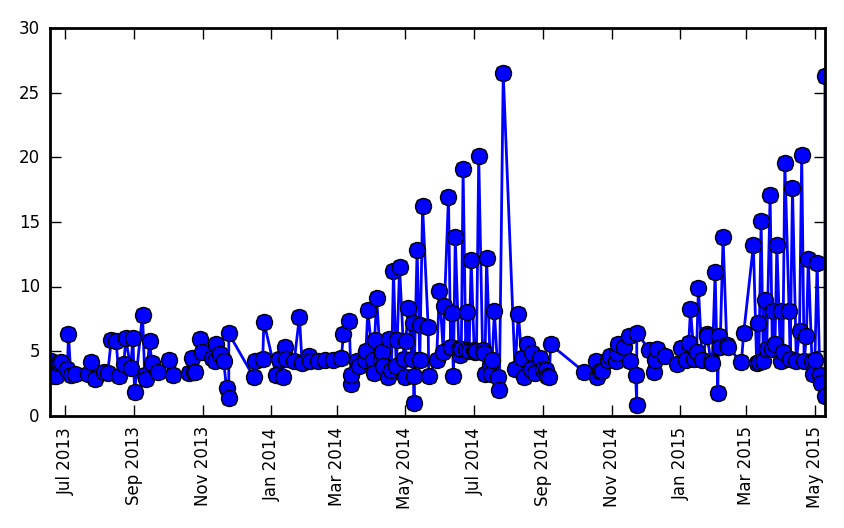I usually run about 4 miles at a time. My two periods of marathon training are pretty obvious on this graph - two 18-week spans where I often went long.

## Cumulative mileage¶

A good way of visualizing cumulative mileage is the famous "Goering diagram", named for its inventor, Andrea Goering.

In :
import datetime

def in_year(date, year):
begin = datetime.datetime(year, 1, 1)
end = datetime.datetime(year + 1, 1, 1)
return (date > begin) & (date < end)

In :
years = (2013, 2014, 2015)

for i,year in enumerate(reversed(years)):
calendar = dates[mask] + i * datetime.timedelta(365)
p.plot(calendar, logged, 'o-', label=str(year))
p.xticks(rotation='vertical')
p.ylabel('cumulative mileage')
p.legend(loc='best');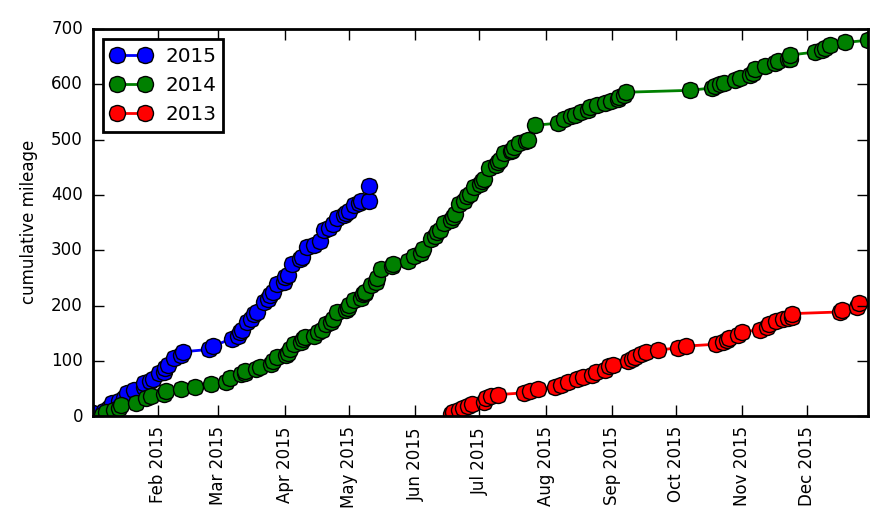It looks like I've really been getting after it in 2015, which is true, but not any more so than in 2014. I've just been doing so earlier in the year, since the marathon was moved from late July to early May.

A fairer Goering diagram would compare my 18-week preparation for each of these races in isolation:

In :
races = [
('EM 2014', datetime.date(2014, 7, 27)),
('EM 2015', datetime.date(2015, 5, 10)),
]

In :
def end_of_day(date):
return datetime.datetime.combine(date, datetime.time(23, 59, 59))

def in_training(date, race_day):
end = end_of_day(race_day)
begin = end - datetime.timedelta( 7 * 18 )  # 18 weeks
return (date > begin) & (date < end)

In :
for year,race_day in reversed(races):
tminus = [dt.days for dt in diff]
p.plot(tminus, logged, 'o-', label=str(year))
p.xticks(rotation='vertical')
p.ylabel('cumulative mileage')
p.xlabel('race countdown')
p.legend(loc='best');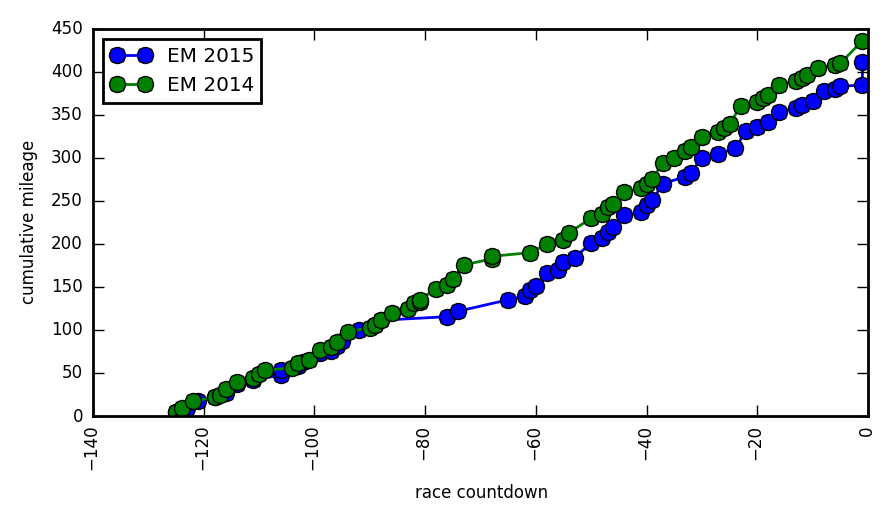I've actually trained less for the 2015 race than I did for the 2014 one. I lost two consecutive weeks to injury/illness this time around, as opposed to only one last year.

Outside of those periods, my training has been more or less identical. I stick with "Novice 2" by Hal Higdon.

# Stream data¶

Strava also provides 'stream data' - raw data logs from the workout. I'll fetch a couple from a track workout I did with the TRE Flyers a couple weeks ago.

In :
FLYERS = 291449438
run = c.get_activity(FLYERS)
run

WARNING:stravalib.model.Activity:No such attribute similar_activities on entity <Activity id=291449438 name=u'Your Fly is Open' resource_state=None>

Out:
<Activity id=291449438 name=u'Your Fly is Open' resource_state=3>
In :
types = ['time', 'moving', 'distance', 'velocity_smooth']

In :
# download streams from strava
streams = c.get_activity_streams(291449438, types)

In :
time =     np.array(streams['time'].data)
distance = np.array(streams['distance'].data)
moving   = np.array(streams['moving'].data)
velocity = np.array(streams['velocity_smooth'].data)


It's easy to plot a pace graph for the workout:

In :
p.plot(time, velocity)
p.xlabel('time (seconds)')
p.ylabel('speed (m/s)')

Out:
<matplotlib.text.Text at 0x10807c310>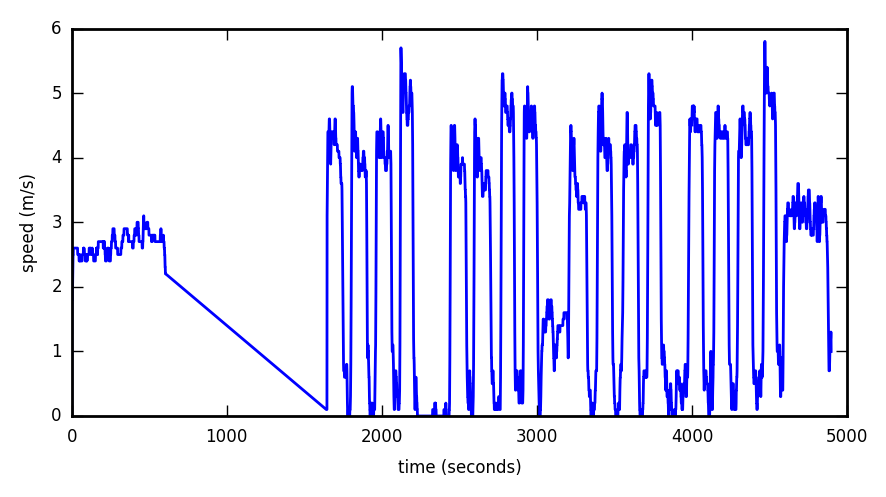Looks like I did 4 sets of 4x400m, with a warmup mile and a couple cooldown laps.

I can also plot cumulative distance within the workout:

In :
p.plot(time, distance, '-o')
p.xlabel('time (seconds)')
p.ylabel('distance (m)')

Out:
<matplotlib.text.Text at 0x10390fcd0>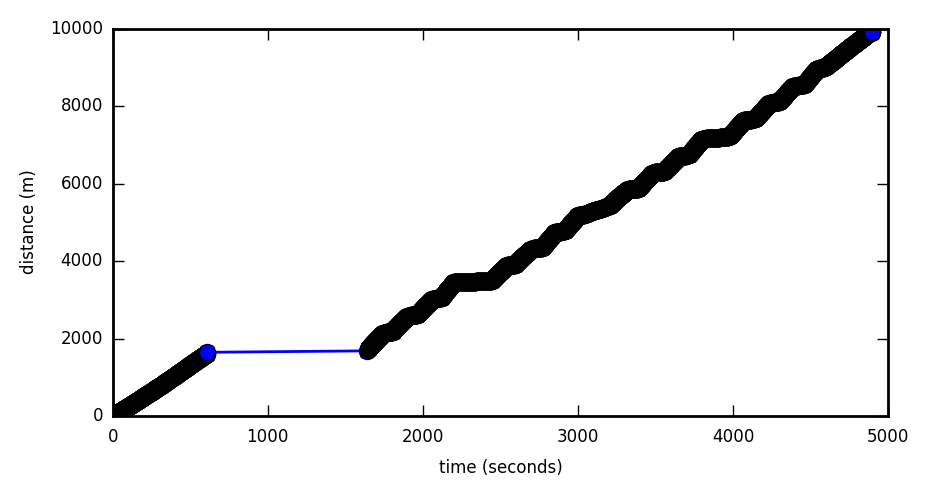I paused my GPS for a while after my warmup lap, but I left it running during the other rests. Strava can figure out when I wasn't moving, regardless of whether I paused the recording. This is the "moving" stream:

In :
p.plot(time[moving], distance[moving], '-o')

Out:
[<matplotlib.lines.Line2D at 0x108707450>]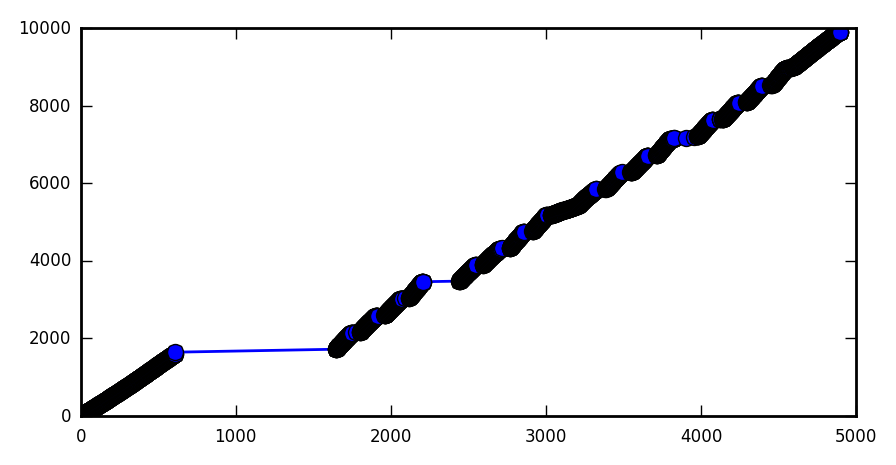# Trajectory plots¶

Now I'll do a cumulative distance plot (trajectory) of each run in the 18-week training period. Hopefully they will scatter promisingly around my target pace.

In :
ids = np.array([a.id for a in runs])

In :
prep = {}
for race,race_day in reversed(races):

In :
prep_2014 = prep['EM 2014']
prep_2015 = prep['EM 2015']


The following two cells make many Strava requests, so I will avoid re-running them.

In :
tracks_2014 = [c.get_activity_streams(run_id, types) for run_id in prep_2014]

In :
tracks_2015 = [c.get_activity_streams(run_id, types) for run_id in prep_2015]

In :
MILE = 1609.
HOUR = 3600.

In :
def make_trajectory(streams):
time =     np.array(streams['time'].data)
distance = np.array(streams['distance'].data)
moving   = np.array(streams['moving'].data)
velocity = np.array(streams['velocity_smooth'].data)

return time[moving], distance[moving]/MILE

In :
def make_trajectory_plot(tracks, highlight_last=True):

for streams in tracks:
t,d = make_trajectory(streams)
p.plot(t/HOUR, d, 'k-')

if highlight_last:
p.gca().lines[-1].set_color('r')
p.gca().lines[-1].set_lw(2)

p.plot([0,4], [0,26.2], 'c--') # goal
p.ylim(0,26.2)
p.xlim(0,4.5);
p.xticks(np.arange(0,5))

In :
f, (ax1, ax2) = p.subplots(1, 2, sharex=True, sharey=True)

p.sca(ax1)
make_trajectory_plot(tracks_2014)
p.sca(ax2)
make_trajectory_plot(tracks_2015)

for ax,label in zip((ax1,ax2), (2014,2015)):
p.text(0.1, 0.8, label, transform=ax.transAxes)
ax.set_xlabel('time (hours)')
ax1.set_ylabel('distance (miles)')

Out:
<matplotlib.text.Text at 0x108994ad0>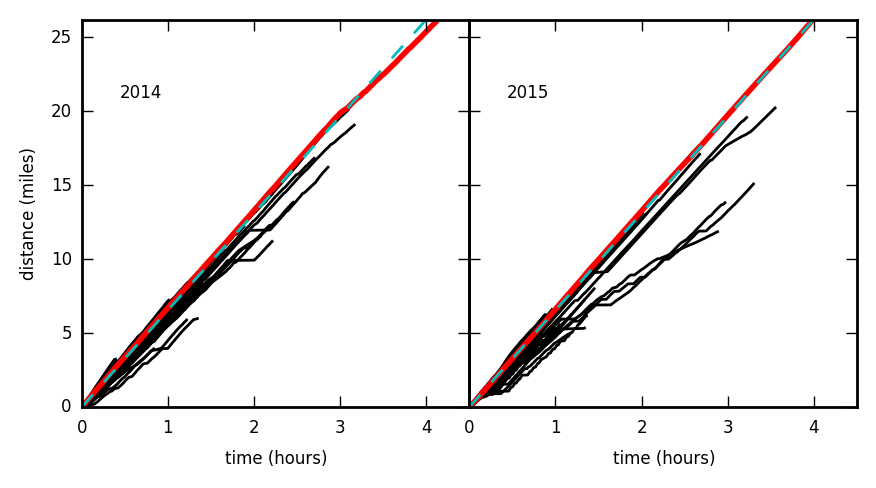There's a lot of information in here, but it's not being represented very well within the rectilinear plot. To use the space more efficiently I'll subtract off my goal pace.

## difference from goal¶

In :
PACE = 4 * 60 / 26.2  # minutes per mile
PACE

Out:
9.160305343511451
In :
def fmt_pace(pace):
minute = pace // 1
second = 60 * (pace % 1)
return "%d:%02d" % (minute, second)

In :
fmt_pace(PACE)

Out:
'9:09'
In :
def add_contours(dmin=-4, dmax=2, mileposts=True, pacegroups=None):
# differential distance, total time
dd = np.linspace(dmin, dmax, 100)
tt =  np.linspace(0, 4.5, 101)

TT,DD = np.meshgrid(tt,dd)
goal_speed = 1/(PACE * 60)
total_distance = (goal_speed * TT * HOUR) + DD

if mileposts:
levels = [26.2, 20, 13.1, 6.2, 3.1]
labels = ['M', '20', 'M/2', '10K', '5K']
cs = p.contour(TT, DD, total_distance, levels=levels, colors='m', linestyles='-')
p.clabel(cs, fmt=dict(zip(levels, labels)))

# avoid dividing by zero
current_pace = (TT * 60) / total_distance

if pacegroups is not None:
cs = p.contour(TT, DD, current_pace, levels=pacegroups, colors='c')
p.clabel(cs, fmt=fmt_pace)

In :
def make_level_trajectory_plot(tracks, highlight_last=True,
mileposts=True,
pacegroups=None,
dlim=(-4,2)):

dmin,dmax = dlim

lines = []
for streams in tracks:
t,d = make_trajectory(streams)
goal_speed = 1/(PACE * 60)  # miles per second (!)
line, = p.plot(t/HOUR, d - t*goal_speed, 'k-')
lines.append(line)

if highlight_last:
p.gca().lines[-1].set_color('r')
p.gca().lines[-1].set_lw(2)

if mileposts or pacegroups:

p.axhline(0, c='c', ls='--') # goal
p.ylim(dmin, dmax)
p.xlim(0,4.5);
p.xticks(np.arange(0,5))

return lines

In :
f, (ax1, ax2) = p.subplots(1, 2, sharex=True, sharey=True)

pacegroups = [7, 8, 8.5, 9, 9.5, 10, 11, 12]

p.sca(ax1)
make_level_trajectory_plot(tracks_2014, pacegroups=pacegroups)
p.sca(ax2)
make_level_trajectory_plot(tracks_2015, pacegroups=pacegroups)

for ax,label in zip((ax1,ax2), (2014,2015)):
p.text(0.05, 0.1, label, transform=ax.transAxes)
ax.set_xlabel('time (hours)')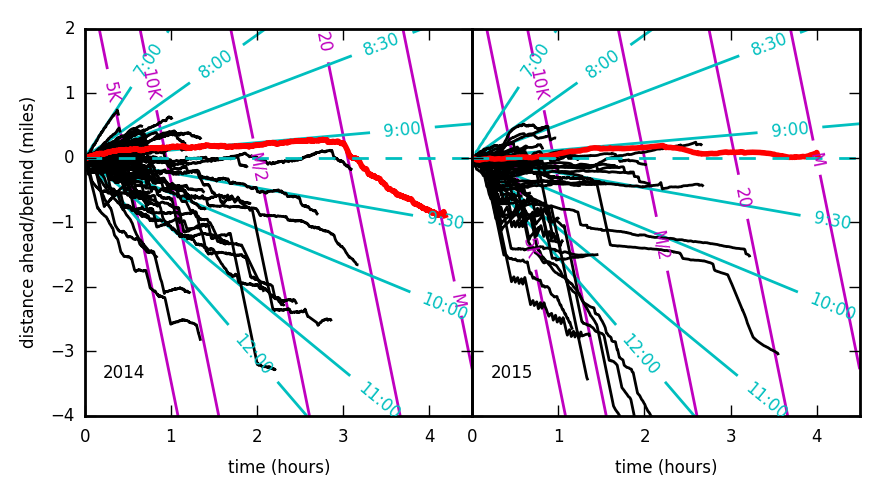From here I can see a lot of detail. Downward-sloping black lines are runs done slower than 4-hour pace, upward-sloping lines are runs done faster than pace. Cyan contours show some other pace trajectories, while magenta contours show distance. The red line is the race.

In last year's marathon, I ran with the CLIF bar 4:00 pacer, who was banking a substantial amount of time early on - he was running at 9:00 even, 9 seconds faster than pace. In addition to the brisk pace, I was fighting some serious cramps and gastrointestinal problems. I lost the pace group at mile 18 and started walking at mile 20. I managed to run most of the last six miles, but I never hit my pace again.

In each training program I did four long runs (between 13 and 20 miles). In 2014 the 20-miler was my best long run (I stayed on pace for it), while in 2015 it was my worst. In both years I had one good long run, one terrible one, and two pretty okay ones. It's not my goal to do every run on pace - in fact, I usually go slower.

One other difference is that I did interval training in 2015. These runs are slow on average, but involve short lengths of fast running (6:30 pace for a few hundred meters). The Flyers workout singled out above is the jagged line that's the lowermost one in 2015.

Overall, I can't say this training cycle is demonstrably stronger than last year's. But I feel pretty good about it.

# Update: 2015 Race Summary¶

In :
g = p.figure()
dlim = (-1,.5)
races = tracks_2014[-1:] + tracks_2015[-1:]
pacegroups = [8.5, 8.75, 9, 9.25, 9.5]
lines = make_level_trajectory_plot(races, dlim=dlim, pacegroups=pacegroups)
p.legend(lines, ('EM 2014', 'EM 2015'))

p.xlabel('time (hours)')

Out:
<matplotlib.text.Text at 0x1072ea510>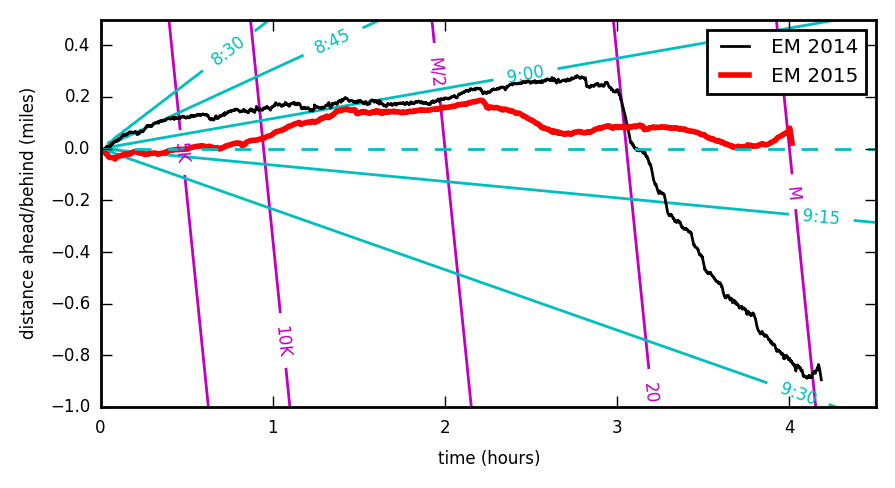I made my 4-hour goal by the skin of my teeth, in 3:59:54. My strategy was to avoid banking time, but I wound up doing so anyway, especially while running through the exciting spectator zones after the 10K mark. At the half marathon I had over a minute put away, but lost it while making friends with another runner in Alton Baker park (I thought I could chat casually and hold my pace, but... I was wrong).

At mile 24 I got swept up by the 4-hour pace group (which, unlike last year, was banking virtually nothing) and they kept me on target for two very difficult miles. When I entered Hayward field and saw less than a minute left on my watch, I drew heavily on my training with the TRE Flyers to finish the last 200 meters with only six seconds to spare.

I had some mild muscle cramps in the second half of the race, but nothing too serious - I think this is very close to the best race I could have run at this level of training. I'm very happy to have made my goal, and am satisfied for now - I think I can go faster, but not without a much more rigorous training load. Many of my friends who ran fast marathons today do 60 miles of training per week, and I never did more than 40.

I'll have a crack at that some other year - in the meantime, it's climbing season.

## A note on the trajectory diagram¶

I like this method of visualizing race progress - It's basically a Goering diagram where slope is normalized to the desired goal. During the race I visualized this in my mind, with a focus on staying above the level as my time ran out. It helped me think about marathoning not as moving fast, but as standing still at 9:09 per mile. In races this long it really is about enduring a pace, not covering a distance.

However, one variation that might be useful is to plot distance as the independent variable, and the difference in time as the dependent one. In this view, trajectories no longer pass the vertical-line test, but they more closely matche race-math mentality: You think of time as what you're banking, not distance.

In :
def add_pace_contours(tmin, tmax, gongstrikes=None, pacegroups=None):
# differential time, total distance
dt = np.linspace(tmin, tmax, 100)
td =  np.linspace(0, 27, 101)

TD,DT = np.meshgrid(td,dt)
elapsed = PACE * TD - DT

if gongstrikes is not None:
levels = [26.2, 20, 13.1, 6.2, 3.1]
cs = p.contour(TD, DT, elapsed/60., levels=gongstrikes, colors='m', linestyles='-')
p.clabel(cs, fmt=fmt_pace)

# avoid dividing by zero
TD[TD <=0 ] = np.nan
current_pace = elapsed / TD
#current_pace = PACE + DT / TD

if pacegroups is not None:
cs = p.contour(TD, DT, current_pace, levels=pacegroups, colors='c')
p.clabel(cs, fmt=fmt_pace)

In :
def make_pace_plot(tracks, highlight_last=True,
gongstrikes=None,
pacegroups=None,
tlim=(-10,4)):

tmin,tmax = tlim

lines = []
for streams in tracks:
t,d = make_trajectory(streams)
goal_pace = (PACE * 60)  # seconds per mile
line, = p.plot(d, -(t - d*goal_pace)/60., 'k-')
lines.append(line)

if highlight_last:
p.gca().lines[-1].set_color('r')
p.gca().lines[-1].set_lw(2)

if (gongstrikes is not None) or (pacegroups is not None):

p.axhline(0, c='c', ls='--') # goal
p.ylim(tmin, tmax)
p.xlim(0,27);
p.xticks([26.2, 20, 13.1, 6.2, 3.1], ['M', '20', 'M/2', '10K', '5K'])

return lines

In :
h = p.figure()
pacegroups = [8.5, 8.75, 9, 9.25, 9.5]
lines = make_pace_plot(races, gongstrikes=np.arange(.5,5,.5), pacegroups=pacegroups)
p.legend(lines, ('EM 2014', 'EM 2015'))
p.xlabel('distance')

Out:
<matplotlib.text.Text at 0x1186df710>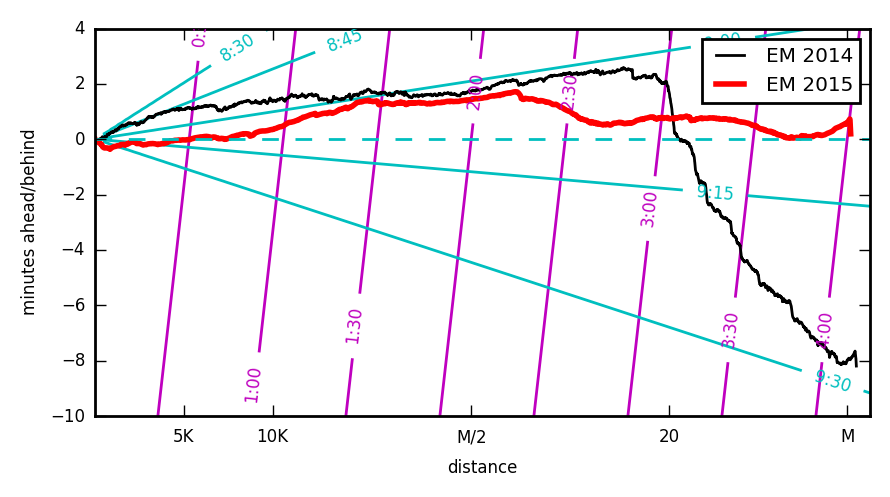Now the magenta contours are elapsed time (since distance is on the axis), but cyan contours are still pace information.

It looks only slightly different, but the Y-axis is actually useful. This makes a couple things clear: for one, I had 2 whole minutes in the bank when things fell apart last year, and that quickly turned into an 8-minute deficit. I never banked that much in 2015, which might have helped me save energy for late in the race - If I hadn't sped up at mile 24, I would have missed the goal.

## A word on GPS accuracy¶

The discrepancy between GPS distance and actual race distance is also evident on this plot. From this diagram you'd think that I finished the 2014 marathon in less than 4:08, but I actually finished in 4:09:50 - nearly off the chart. At that time I was using my iPhone 4S to record activities, which is not very accurate. Strava thinks I finished the marathon 2 minutes before I actually did, because my phone overestimated the distance I was covering.

This is a less serious problem now that I use the TomTom Runner watch, but it's still there. From this plot it looks like I finished the race with more than 30 seconds to spare (ignore the sharp dip at the end - that's me letting my watch run an extra 15 seconds post-finish). In fact it was only 6. GPS error contributes to that, and other things (like going wide around a corner) probably do too.

This is why Strava lists achievements as "estimated best effort" - it really is just an estimate. My marathon EBE is now listed as 3:59:24 - when my actual time is 3:59:54. So the TomTom exaggerates my performance by 30 seconds as opposed to 2 whole minutes with the iPhone. That's a big difference, and I'm really glad I switched.

In :
g.savefig('racecompare.jpg', dpi=600, quality=95)
g.savefig('racecompare.pdf')

In :
h.savefig('pacecompare.jpg', dpi=600, quality=95)
h.savefig('pacecompare.pdf')

In :
f.savefig('2marathons.jpg', dpi=600, quality=95)

In :
g.savefig('racecompare.jpg', dpi=600, quality=95)
g.savefig('racecompare.pdf')

In :
f.savefig('2marathons.pdf', dpi=600)